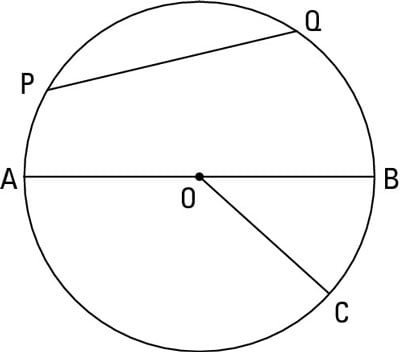##### Geometry For DummiesWhen you’re working with a circle, there are five important theorems that you need to know about the properties of the circle’s radii and chords. (There are really just three ideas; but two of the theorems work in both directions which brings the total to five):Segment PG is a chord, segment AB is the diameter, and segment OC is the radius of this circle.

• Perpendicularity and bisected chords:

• If a radius is perpendicular to a chord, then it bisects the chord.

• If a radius bisects a chord (that isn’t a diameter), then it’s perpendicular to the chord.

• Distance and chord size:

• If two chords of a circle are equidistant from the center of the circle, then they’re congruent.

• If two chords of a circle are congruent, then they’re equidistant from its center.